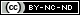## Reinventing The Wheel II

16 04 2011flickr: by kevinspencer

If you’re curious about how to merge two unsorted and mixed value arrays into one that is in ascending order, an idea I posed in the previous post Reinventing The Wheel, here’s the algorithmic code, including benchmarking:

```\$arr1 = array('X','p','d','2','7','3','x');
\$arr2 = array('44','h','66','B','0','w','A');

// merging without array_merge()
function arrMerge(&\$arr1, \$arr2)
{
\$count = getCount(\$arr2);
for(\$i=0; \$i < \$count; \$i++) {
\$arr1[] = \$arr2[\$i];
}
}

// filtering without array_filter()
function getValues( \$arr)
{
\$temp = null;
\$temp2 = null;
\$count = getCount( \$arr );
for( \$i=0; \$i < \$count; \$i++) {
\$value = \$arr[\$i];
if (\$value == '0') {
\$temp2[] = \$value; // store in numbers array
}
else
if (\$value == 0) {
\$temp[] = \$value; // store in letters array
}
else
{
\$temp2[] = \$value;  // store in numbers array
}
}
return array(doSort(\$temp),doSort(\$temp2));
}

function getCount( \$arr ) // counting minus count()
{
\$count=0;
foreach(\$arr as \$element) {
\$count++;
}
return \$count;
}

// sorting without sort()
function doSort( \$arr )
{
\$count = getCount(\$arr);

for (\$i=0; \$i < \$count; \$i++){
for (\$j=\$i; \$j < \$count; \$j++) {
\$placeholder = '';
if (  \$arr[\$i] > \$arr[\$j] ) {
\$placeholder = \$arr[\$i]; // save it
\$arr[\$i] = \$arr[\$j];
\$arr[\$j] = \$placeholder;
}// end if
}	// end inner for
}// end outer for
return \$arr;
}
\$start = microtime();
arrMerge(\$arr1, \$arr2);
\$multiDim = getValues( \$arr1 );

arrMerge(\$multiDim,\$multiDim);

\$end = microtime();
\$time = \$end - \$start;
var_dump(\$multiDim);
echo \$time;
```

Initially, I tried this code without using any user-defined functions. But, modularity is important, too, so I’ve put in a few user-defined functions to keep the code somewhat organized and readable. I really wanted to use the built-in function ord() to differentiate between the character and numeric data.  After the code was working with it, I realized I could get by without it. Strings with just letters have a numeric value of zero but are not identical to zero. So I take advantage of that fact to separate the numeric and character data into two separate arrays such that each comprises an element of  array \$multiDim.  And, yes, the code does seem to run faster without ord().

This work is licensed under a### Information

This site uses Akismet to reduce spam. Learn how your comment data is processed.